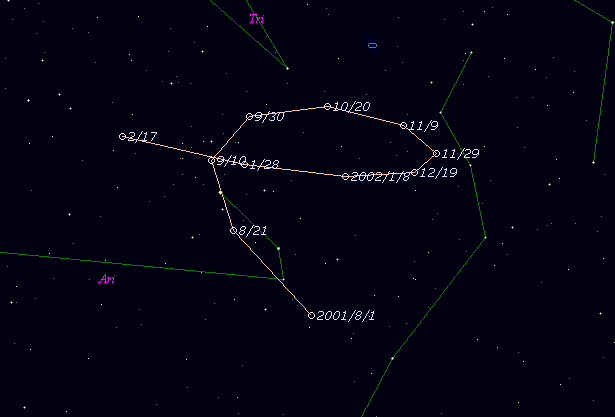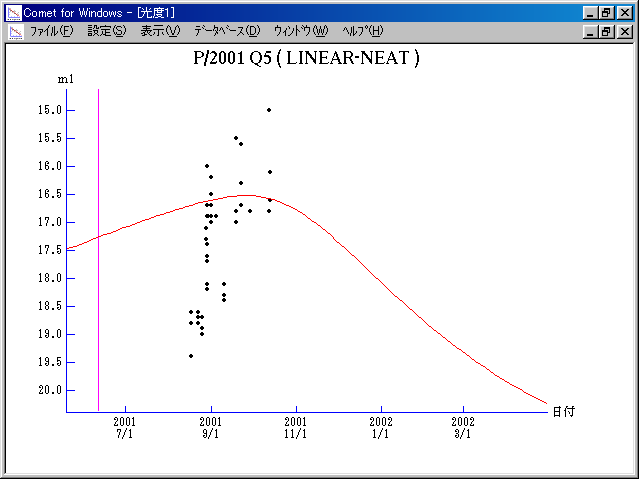# \$B%j%K%"(B-\$B%K!<%HWB@1(B

193P/LINEAR-NEAT (2001)###\$B%W%m%U%#!<%k(B

 \$BId9f(B 193P/2001 Q5 \$BH/8+F|(B 2001\$BG/(B8\$B7n(B17\$BF|(B, 8\$B7n(B28\$BF|(B \$BH/8+8wEY(B 17.3\$BEy(B \$BH/8+ Lincoln Laboratory Near-Earth Asteroid Research project, Near-Earth-Asteroid Tracking Team (Jet Propulsion Laboratory)

###\$B50F;MWAG(B

```                    Epoch = 2001 June 20.0 TT
T = 2001 June 11.6210 TT         Peri. =   6.3996
e = 0.416559                     Node  = 336.2663  2000.0
q = 2.043148 AU                  Incl. =  10.9448
a =  3.501896 AU    n = 0.1504005    P =   6.553 years
```

###\$B@1?^(B###\$B8wEYJQ2=(B

```        m1 = 12.5 + 5 log\$B&\$(B + 10.0 log r
```##### \$B50F;MWAG\$O!"(BIAUC 8885\$B\$K7G:\\$5\$l\$?\$b\$N\$G\$9!#(B \$B@1?^\$O(B StellaNavigator Ver.2.0 for Windows (\$B%"%9%H%m%"!<%D(B \$BJTCx(B / \$B%"%9%-!<=PHG6I4)(B) \$B\$G:n@.\$7\$?\$b\$N\$G\$9!#(B \$B8wEY%0%i%U\$O(BComet for Windows\$B\$G:n@.\$7\$?\$b\$N\$G\$9!#(B